# Excel 2010: Matching Values With EXACT Function

Excel 2010 provide an easy way to find out the matching value between multiple fields. Through EXACT function you will be able to find out the exact match between two strings, it takes two argument to be matched against each other and return results in logical value either TRUE or FALSE, so you can easily categorize the match field by applying desired functions and formulas over two logical values. The EXACT function is case-sensitive, i.e, it returns TRUE only if case of both strings are same.

Open Excel 2010 spreadsheet on which you want to apply EXACT function, for instance we have included a spreadsheet containing fields; Name and Course.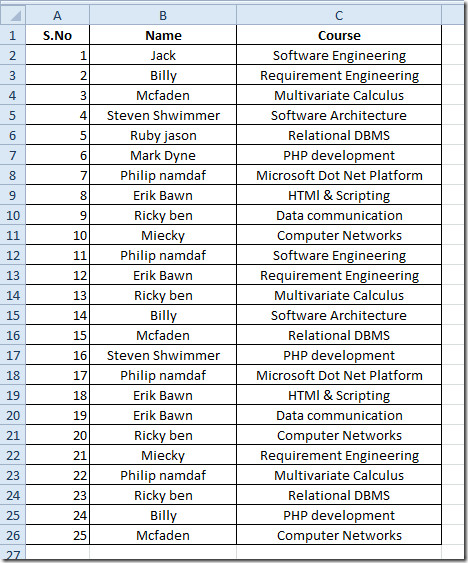Now if you need to match the Name field with some other field seemingly contains same data value, the EXACT function would be useful. We have another field by the name of Students for which we need to find out the discrepancy between two fields.

The syntax of EXACT function is;

=EXACT(tex1,text2)

The function arguments text1 and text2 are two strings which can be entered directly or you can provide reference cell location, where the string is residing.

We will be checking for exact match in the field next to Students, as shown in the screen shot below.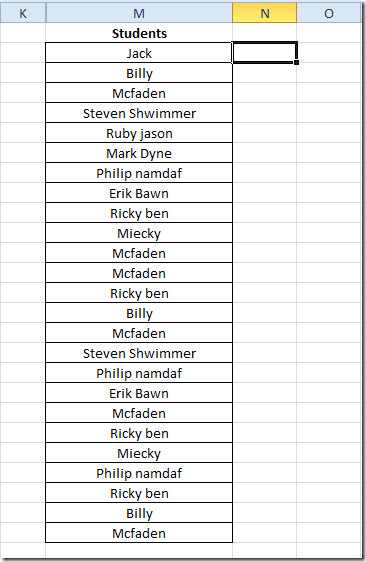We will write this function as;

=EXACT(B2,M2)

In function arguments, B2 is the location of first cell in Name field and M2 is the location of cell in Students field. It will yield logical value, i.e, TRUE for exact match and FALSE for converse result.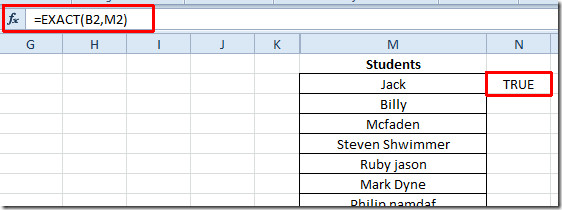Now drag the plus sign at the end of the cell towards the end of the column for checking exact matches. It will show FALSE where the strings do not match with each other.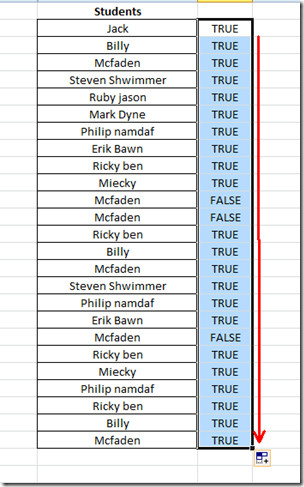If you want to check the exact match by putting values directly, then you need to enter values in double quotations marks.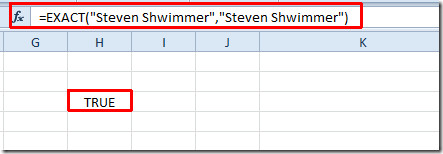You can also check out previously reviewed Excel function; MAX,MIN, MAXA, MINA and Logical Functions, INFO, SUMSQ, DOLLAR, SUMPRODUCT, SUMIF, COUNTIF, VLOOKUP, HLOOKUP ,PMT, & LEN.

1.Tazuddin says:
2.Monica says: